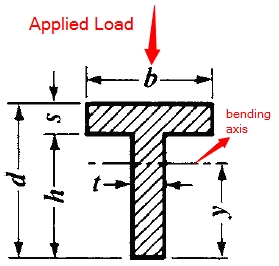This is the T Beam Moment of Inertia Calculator. Simply enter the dimensions below for T beam to find the moment of inertia.d = h = b = t = s =